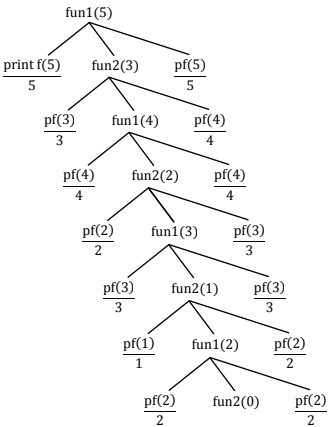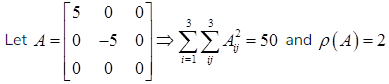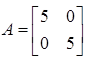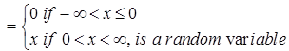# GATE Solved Paper 2017-19 - GATE 2017 Shift 1

>>>>>>>>GATE 2017 Shift 1

• A

53423122233445• B

53423120112233• C

53423122132435• D

53423120213243• Option : A
• Explanation :Consider the following statements.
(I) One eigen value must be in [-5, 5]
(II) The eigen value with the largest magnitude must be strictly greater than 5.
Which of the above statements about eigen values of A is/are necessarily CORRECT?

• A

Both (I) and (II)• B

(I) only• C

(II) only• D

Neither (I) nor (II)• Option : B
• Explanation :
ρ(A) = n|A| = 0 ⇒ one eigen value must be ‘0’ ∈ [-5, 5]
∴ (I) is truebut eigen values of A are 0, −5,5
∴ The eigen value with the largest magnitude is not greater than 5
For and Let⇒ eigen values = 5,5
∴ One eigen value must be in [−5,5] and largest eigen value magnitude is not greater than 5
∴ (II) is false

• A

Finite• B

Not finite but regular• C

Context-free but not regular• D

Recursive but not context-free• Option : B
• Explanation :
The Context free grammar given over alphabets ∑{a, b, c} with S and T as non terminals are:
G1: S → aSb|T,T → cT|∈
G2: S → bSa|T,T → cT|∈
Lets L(G1) is the language for grammar G1 and L(G2) is the language for grammar G2
L(G1) = {ancmbn|n,m ≥ 0}
L(G2) = {bncman|n,m ≥ 0}
L(G1) ∩ L(G1) = {cman|m ≥ 0}; which is infinite and regular

• A

0• B

1• C

2• D

3• Option : A
• Explanation :
‘X’ is Gaussian random variable
⇒ X ∼ N(0, σ2) for -∞ < x < ∞
GivenSince median is positional average
Therefore, median of Y is '0'.

Assume for all inputs (a, b, c, d) the respective complements (a, b, c, d) are also available. The above logic is implemented 2-input NOR gates only. The minimum number of NOR gates required is _____.

Note – Numerical Type question

• A

0• B

1• C

2• D

3• Option : B
• Explanation :
From K-map simplification we get the min-term as CA' . So We can simplify it for NOR gate expression
i.e. C' NOR A = (C'+A)' = CA'
Now complemented inputs are also given to us so for 2 input NOR gate we need only 1 NOR gate.
1 is correct answer
Related Quiz.
GATE 2017 Shift 1